# IoT Security – Part 22 (Blind Signal Analysis using Python)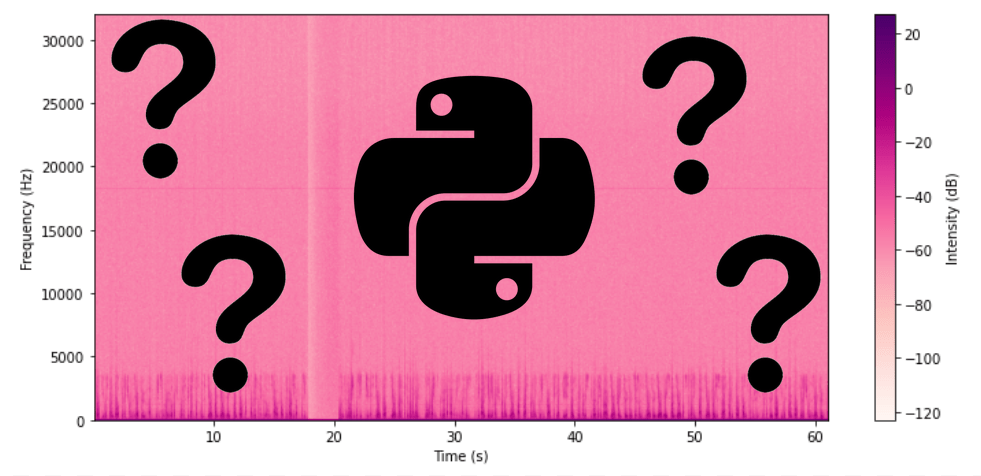## Introduction

This blog is part of the IoT Security series, where we discuss the basic concepts about the IoT/IIoT ecosystem and its security. If you have not gone through the previous blogs in the series, I will urge you to go through those first. In case you are only interested in signal processing, feel free to continue.

IoT Security – Part 1 (101 – IoT Introduction And Architecture)

IoT Security – Part 21 (Famous IoT Attacks & Vulnerabilities) previous blog in the series.

Let’s take a scenario where the you are able to capture an unidentified signal, this includes the audio as well the raw iq format of the signal. What should be our approach to get the maximum information from that signal. So digging into the properties of the signal should be our first approach which is also know as blind signal analysis, and by that we mean identifying it’s properties like frequency, amplitude and phase of the signal et cetra

So again the question might arise, why should we care about these parameters? And the simple answer to this is because these are the parameters that actually change in the signal when some information has to be communicated i.e. voice, data etc.

In this blog we will be performing basic blind signal processing techniques using python in order to get more out of an unknown signal.

## Capturing the signal

For this blog, we’ll be focusing more on the .wav capture (audio) and in next part we will work around on the raw iq samples.

Quick tip: Always make sure that raw signal is not just captured in audio format (.wav) but also in I/Q form (.cu8 or .bin) to ensure no insights on the signals are missed.

You can try the following with any capture via your SDR, for this demonstration we are using a raw signal from the Sigidwiki

Let’s not reveal what signal did we pick, we will find it out on the way!

For our signal processing we will require a python3 environment.

``````1import matplotlib.pyplot as plt # to plot our outputs
2import numpy as np # to import numpy
3from scipy import signal # for signal processing
4from scipy.io import wavfile # to analyse the .wav file
5from numpy import fft as fft # to calculate fft
6sample_rate, samples = wavfile.read('AM_IQ.wav') # gives sample rate and data from the .wav file
7
``````

These are a few libraries we require in order to start with our analysis.

## Frequency and sampling rate

In most cases, a recorded signal will come with a tag of the frequency at which it is recorded.

One can assume that at least twice of this frequency is the minimum sampling frequency (nyquist sampling theorem) but we can’t be sure as the wav files received which are rationally resampled, that is the reason why we will be splitting our signal into two seperate channels as we move forward.

In order to get the sampling rate and number of channels:

``````1print (sample_rate,"is the sampling rate")
2print("The channel count of the input wav file is", np.shape(samples))
``````

Output:

``````164000 is the sampling rate
2The channel count of the input wav file is 2
``````

So it comes out that our signal was sampled at 64KHz and we have two channels in our raw I/Q signal.

## Data type of the captured file

There are numerous data types like uint8, int 16, float 32, complex 32 etc.

Now you must be wondering how does that aid in our signal analysis?

The simple answer to this is it can tell us about the Analog-to-Digital Converter (ADC) used and possibly about the SDR peripheral device (frontend) being used to capture the signal.

``````1filetype = samples.dtype
2print(filetype)
``````

Output:

``````1uint8
``````

So our signal comes out to be an unsigned integer of 8 bits. Now we can easily look up the ADC and their output types amongst the popular SDR and based on that we can say that this signal was most probably captured using a hackrf one.

## Splitting the channels

As we know that a raw signal is made up of two channels one is the I(inphase) channel and other is the Q(quadrature) channel. So now we will split our captured signal into two channels.

c1 is the Inphase part and c2 is the Quadrature part of the signal.

``````1c1 = samples[:, 0] #Chan1/ I channel
2c2 = samples[:, 1] #Chan2/ Q channel
``````

## Signal to Noise Ratio (SNR)

If you recall your Signal processing lectures, you would have come across this term most of the time.Remember?

Signal to noise ratio (SNR or S/N) is the ratio between the desired information (actual signal) and the undesired signal (noise). Now we will be calculating the loudness of the audio recorded.

Note: This is not exactly the SNR value of the signal but the loudness parameter and can be used as a ball-park measure of SNR.

``````1snr =(np.sum(c1.astype(np.float)**2))
2print(snr)
``````

Output:

``````164761741609.0
``````

So in our case since we don’t have the base value to calculate the exact SNR, we can say higher the value, the more is SNR and better is the quality of signal.

## Energy per unit time

In a nutshell, energy per unit time of a signal gives us the strength of the signal which is in Joules. It is quite similar to RSSI value in case of Zigbee and WiFi.

``````1en = 1.0/(2*(c1.size)+1)*np.sum(c1.astype(float)**2)/sample_rate
2print(en)
``````

Output:

``````10.1293352042703946
``````

The higher the value, the more the receiver is closer to the source.

## Time vs Amplitude

Time vs Amplitude gives us a visualisation of how the signal’s amplitude changes with respect to the time

`````` 1# scaling the samples wrt to sampling rate time scaled samples are spread on time
2time = np.arange(0, float(samples.shape), 1) / sample_rate # create time variable in seconds
3print(time)
4#plot the dual channel output
5plt.figure(figsize=(10,10))
6# to initialise an empty space and define the dimensions
7plt.subplot(211)
8# for the first channel (I channel)
9plt.plot(time, c1, linewidth=1, alpha=0.9)
10plt.xlabel('Time (s)')
11plt.ylabel('Amplitude')
12plt.subplot(212)
13# for the second channel (Q channel)
14plt.plot(time, c2, linewidth=1, alpha=0.9)
15plt.xlabel('Time (s)')
16plt.ylabel('Amplitude')
17plt.show()
``````

Output: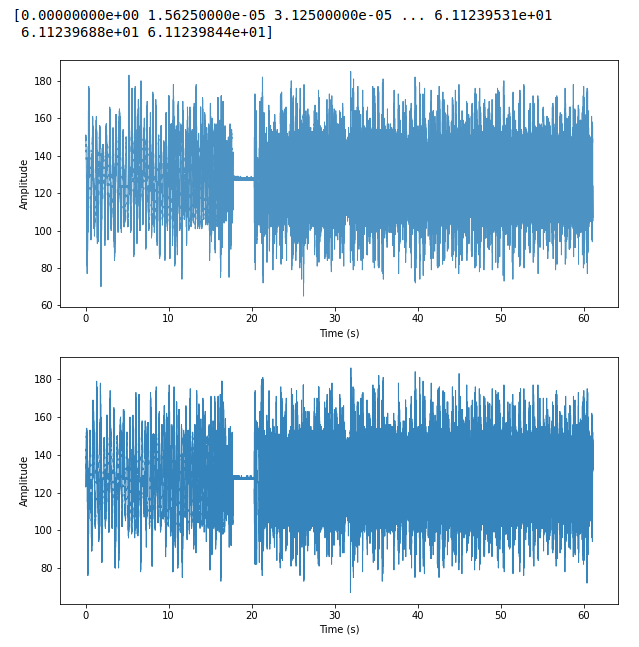The upper plot is for Inphase channel and the lower is for Quadrature channel

Since amplitude vs time for both i and q channels is almost the same, this signifies that our signal could be an amplitude modulated signal.

Further we will be performing the operations on the channel 2 which is the Q channel from the captured audio file

## Fast Fourier Transform (FFT)

Fast Fourier Transform (FFT) as we know can be used to simply characterize the magnitude and phase of a signal, or it can be used in combination with other operations to perform more involved computations such as convolution or correlation. Now let’s take a look at the fft of the channel 2.

``````1fourier = fft.fft(c2)
2plt.plot(fourier)
3plt.xlabel('k')
4plt.ylabel('Amplitude')
5print(fourier)
6print(len(fourier))
``````

output:## Power vs Frequency

The power vs frequency plot is another major observation that we usually do while performing signal processing. The plot gives us an idea of the frequency and their respective power, this helps us find the most relevant spike as that would be the frequency where there is maximum power.

`````` 1n = len(c2)
2#getting length of channel
3print(n)
4l = np.int(np.ceil(n/2))
5print(l)
6#scale the signal and align for magnitude
7fourier = fourier[0:l-1]
8fourier = fourier / float(n)
9freqArray = np.arange(0, l-1, 1.0) * (sample_rate*1.0/n)
10print(np.shape(fourier))
11print(np.shape(freqArray))
12plt.figure(figsize=(5,5))
13plt.plot(freqArray/1000, 10*np.log10(fourier)) # for normalisation in (dB scale)
14plt.xlabel('Frequency (kHz)')
15plt.ylabel('Power (dB)')
``````

output: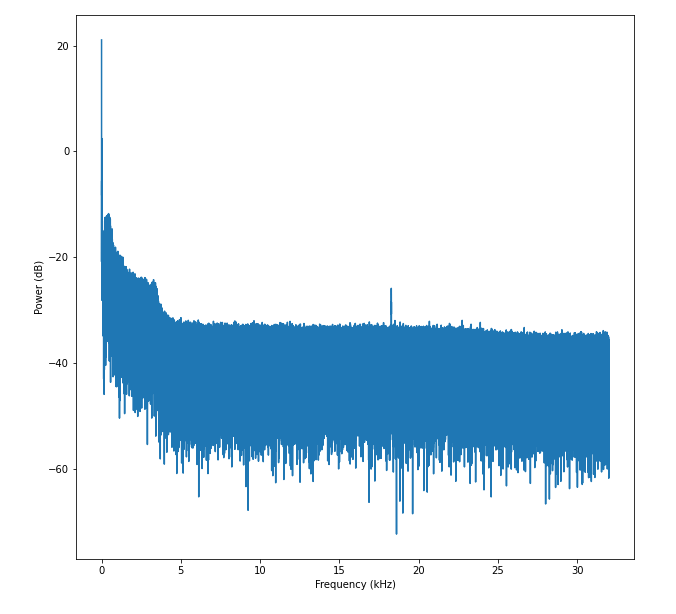## Spectrogram

A spectrogram can be defined as a visual way of representing the signal strength over time at various frequencies present in a particular waveform. Not only does it tell at what frequency there is more or less energy, for example, 2 Hz vs 10 Hz, but one can also see how energy levels vary over time.

``````1plt.figure(2, figsize=(7, 7))
2plt.subplot(211)
3Pxx, freqs, bins, im = plt.specgram(c2, Fs=sample_rate, NFFT=2048, cmap=plt.get_cmap('RdPu'))
4cbar=plt.colorbar(im)
5plt.xlabel('Time (s)')
6plt.ylabel('Frequency (Hz)')
7cbar.set_label('Intensity (dB)')
``````

output:From the graph you can observe that for all the various frequencies the spectrogram remains the same, which means it is frequency invariant. Hence, we can establish the fact that the signal is an Amplitude modulated signal.

## Surfing other frequencies

Just in case, if there are other parts of the signal at different frequencies that we might want to look through for our analysis.

``````1np.where(freqs==10000)
2Miss = Pxx[500, :]
3plt.plot(bins, Miss)
``````

output:## Other channel

We can run the similar analysis on the other channel (our channel 1 which is the I channel) in order to either get more insights or establish our findings from the first channel.

So, by running similar analysis on the I channel we get: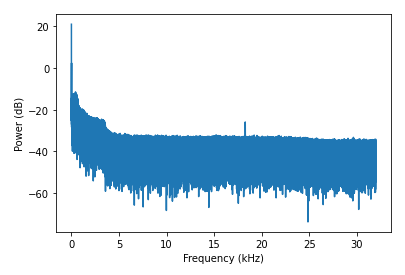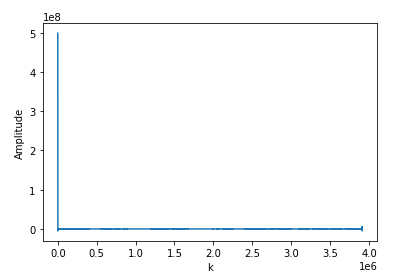What we are trying to imply here is the modulation scheme used in the signal from the two spectrogram plots (I and Q) is Amplitude modulation. We inferred this by visually looking at the spectrogram plots which have peaks in intensity wrt to time at particular frequencies in our case.

## Conclusion

We can conclude that the unknown signal was actually an Amplitude Modulated IQ signal. We went through how just by using python we can perform a blind signal analysis on an unknown signal, all by observing the signal and taking in account the properties of the signal. If you wish to try out a signal analysis on an unknown signal yourself be it digital or analog, you can do that by following the above steps. Try out different signals and observe how the properties vary from signal to signal.

Fill in your details and get your copy of the datasheet in few seconds

CTI Report

Fill in your details and get your copy of the ebook in few seconds

Fill in your details and get your copy of sample report in few seconds

Fill in your details and get your copy of sample report in few seconds

Fill in your details and get your copy of sample report in few seconds

Fill in your details and get your copy of sample report in few seconds

Fill in your details and get your copy of sample report in few seconds

Fill in your details and get your copy of sample report in few seconds

Fill in your details and get your copy of sample report in few seconds

Fill in your details and get your copy of sample report in few seconds

Fill in your details and get your copy of sample report in few seconds

Fill in your details and get your copy of sample report in few seconds